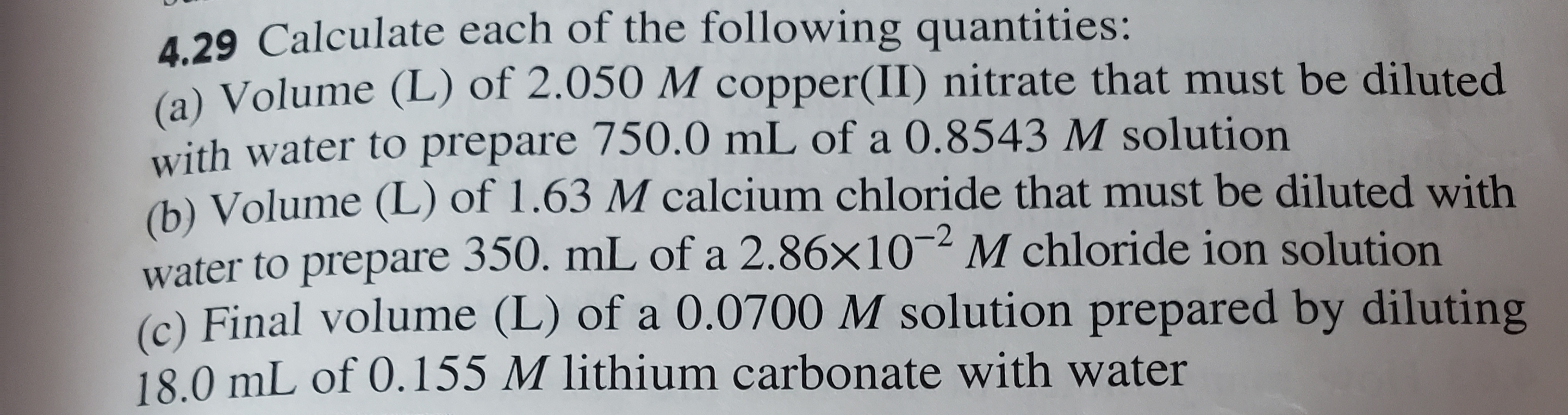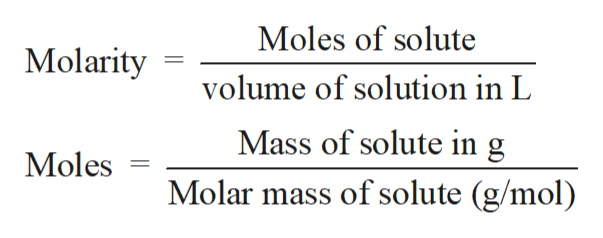4.29 Calculate each of the following quantities:(a) Volume (L) of 2.050 M copper(II) nitrate that must be dilutedwith water to prepare 750.0 mL of a 0.8543 M solution)Volume (L) of 1.63 M calcium chloride that must be diluted withwater to prepare 350. mL of a 2.86x10 M chloride ion solution(c) Final volume (L) of a 0.0700 M solution prepared by diluting18.0 mL of 0.155 M lithium carbonate with water

Questionhelp_outlineImage Transcriptionclose4.29 Calculate each of the following quantities: (a) Volume (L) of 2.050 M copper(II) nitrate that must be diluted with water to prepare 750.0 mL of a 0.8543 M solution )Volume (L) of 1.63 M calcium chloride that must be diluted with water to prepare 350. mL of a 2.86x10 M chloride ion solution (c) Final volume (L) of a 0.0700 M solution prepared by diluting 18.0 mL of 0.155 M lithium carbonate with water fullscreen
Step 1

Solution stoichiometry involves the calculation of concentration of solutions in the given conditions of volumes, moles etc.

Step 2

Solution stoichiometry is mainly based on the calculation of moles and volumes. These two values are used to calculate the molarity of solution. The relation between moles, volume and molarity is as given below;help_outlineImage TranscriptioncloseMoles of solute Molarity volume of solution in L Mass of solute in g Moles Molar mass of solute (g/mol) fullscreen
Step 3

To calculate the molarity or volume of solution after dilution;...

Want to see the full answer?

See Solution

Want to see this answer and more?

Our solutions are written by experts, many with advanced degrees, and available 24/7

See Solution
Tagged in

Chemistry